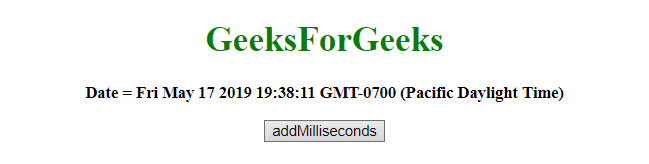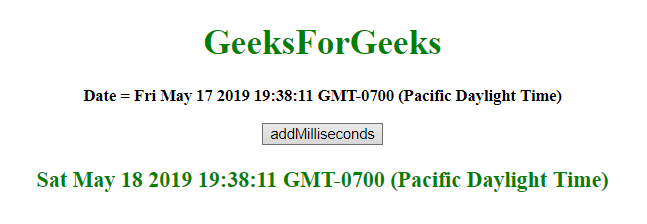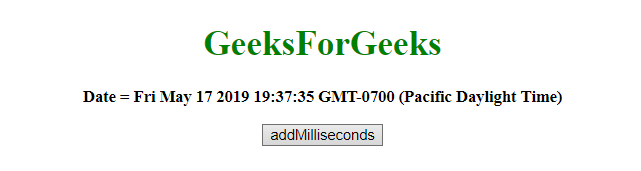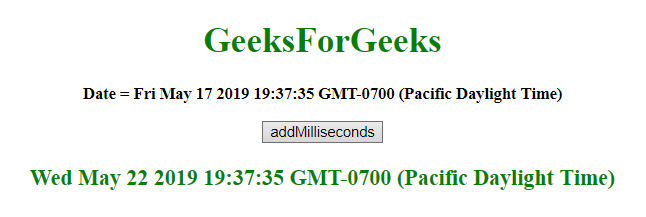# JavaScript | Adding days in milliseconds to Date object

Given a date, the task is to add days in milliseconds to it. To add days in milliseconds to date object in JavaScript, some methods are used which are listed below:

• JavaScript getMilliseconds() Method: This method returns the milliseconds (from 0 to 999) of the provided date and time.

Syntax:

```Date.getMilliseconds()
```

Return value: It returns a number, from 0 to 009, representing the milliseconds.

• JavaScript setMilliseconds() Method: This method sets the milliseconds of a date object.
Syntax:

```Date.setMilliseconds(millisec)
```

Parameters: It accepts single parameter millisec which is required. It specifies the integer value representing the milliseconds. The expected values are 0-999, but other values are allowed.

Note: The parameter accepts values apart from their range and these values works like:

• millisec = -1, it means the last millisecond of the previous second and same for the other parameters.
• If millisec passed as 1000, it means the first millisecond of the next second and same for the other parameters.

Return value: It returns a number, denoting the number of milliseconds between the date object and midnight January 1 1970.

• JavaScript getTime() method: This method returns the number of milliseconds between midnight of January 1, 1970, and the specified date.

Syntax:

`Date.getTime()`

Return value: It returns a number, representing the number of milliseconds since midnight January 1, 1970.

• JavaScript setTime() method: This method sets the date and time by adding/subtracting a defines number of milliseconds to/from midnight January 1, 1970.

Syntax:

`Date.setTime(millisec)`

Parameters: It accepts single parameter millisec which is required. It specifies the number of milliseconds to be added/subtracted, in midnight January 1, 1970

Return value: It returns the number of milliseconds between the date object and midnight January 1 1970.

• Example 1: This example added 1 day in milliseconds to the var today by using setTime() and getTime() method.

 `  ` `<``html``>  ` `    ``<``head``>  ` `        ``<``title``>  ` `            ``JavaScript | Adding days in milliseconds to Date object ` `        `` ` `    ``  ` `     `  `    ``<``body` `style` `= ``"text-align:center;"``>  ` `         `  `        ``<``h1` `style` `= ``"color:green;"` `>  ` `            ``GeeksForGeeks  ` `        `` ` `         `  `        ``<``p` `id` `= ``"GFG_UP"` `style` `= ``"font-size: 15px; font-weight: bold;"``> ` `        `` ` `         `  `        ``<``button` `onclick` `= ``"gfg_Run()"``>  ` `            ``addMilliseconds ` `        `` ` `         `  `        ``<``p` `id` `= ``"GFG_DOWN"` `style` `=  ` `            ``"color:green; font-size: 20px; font-weight: bold;"``> ` `        `` ` `         `  `        ``<``script``> ` `            ``var el_up = document.getElementById("GFG_UP"); ` `            ``var el_down = document.getElementById("GFG_DOWN"); ` `            ``var today = new Date(); ` `            ``el_up.innerHTML = "Date = " + today; ` `             `  `            ``Date.prototype.addMillisecs = function(d) {  ` `                ``this.setTime(this.getTime() + (d));  ` `                ``return this;  ` `            ``} ` `             `  `            ``function gfg_Run() { ` `                ``var a = new Date(); ` `                ``var d = 1; ` `                ``a.addMillisecs(d*24*60*60*1000); ` `                ``el_down.innerHTML = a; ` `            ``}          ` `        ``  ` `    ``  ` `                     `

Output:

• Before clicking on the button:• After clicking on the button:Example 2: This example added 5 days in milliseconds to the var today by using setMilliseconds() and getMilliseconds() method.

 `  ` `<``html``>  ` `    ``<``head``>  ` `        ``<``title``>  ` `            ``JavaScript | Adding days in milliseconds to Date object ` `        `` ` `    ``  ` `     `  `    ``<``body` `style` `= ``"text-align:center;"``>  ` `     `  `        ``<``h1` `style` `= ``"color:green;"` `>  ` `            ``GeeksForGeeks  ` `        `` ` `         `  `        ``<``p` `id` `= ``"GFG_UP"` `style` `= ``"font-size: 15px; font-weight: bold;"``> ` `        `` ` `         `  `        ``<``button` `onclick` `= ``"gfg_Run()"``>  ` `            ``addMilliseconds ` `        `` ` `         `  `        ``<``p` `id` `= ``"GFG_DOWN"` `style` `= ` `            ``"color:green; font-size: 20px; font-weight: bold;"``> ` `        `` ` `         `  `        ``<``script``> ` `            ``var el_up = document.getElementById("GFG_UP"); ` `            ``var el_down = document.getElementById("GFG_DOWN"); ` `            ``var today = new Date(); ` `            ``el_up.innerHTML = "Date = " + today; ` `             `  `            ``Date.prototype.addMillisecs= function(s) { ` `                ``this.setMilliseconds(this.getMilliseconds()+s); ` `                ``return this; ` `            ``} ` `             `  `            ``function gfg_Run() { ` `                ``var a = new Date(); ` `                ``var d = 5; ` `                ``a.addMillisecs(d*24*60*60*1000); ` `                ``el_down.innerHTML = a; ` `            ``}          ` `        ``  ` `    ``  ` `                     `

Output:

• Before clicking on the button:• After clicking on the button:My Personal Notes arrow_drop_upCheck out this Author's contributed articles.

If you like GeeksforGeeks and would like to contribute, you can also write an article using contribute.geeksforgeeks.org or mail your article to contribute@geeksforgeeks.org. See your article appearing on the GeeksforGeeks main page and help other Geeks.

Please Improve this article if you find anything incorrect by clicking on the "Improve Article" button below.